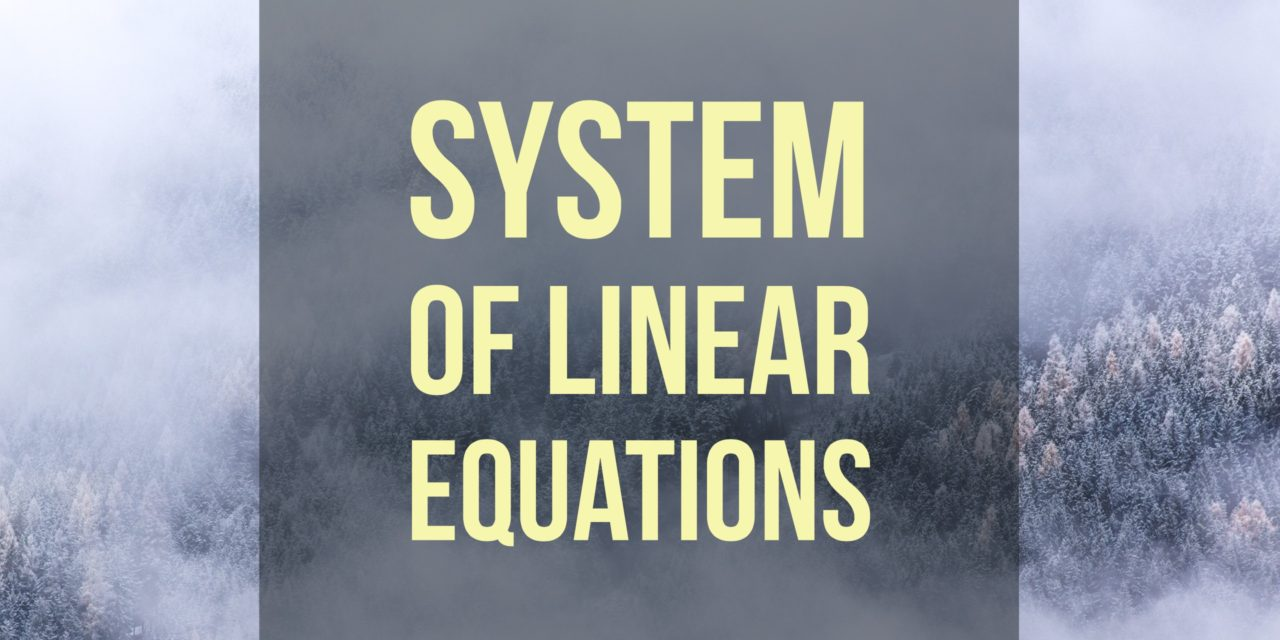# Quiz 4: Inverse Matrix/ Nonsingular Matrix Satisfying a Relation## Problem 289

(a) Find the inverse matrix of
$A=\begin{bmatrix} 1 & 0 & 1 \\ 1 &0 &0 \\ 2 & 1 & 1 \end{bmatrix}$ if it exists. If you think there is no inverse matrix of $A$, then give a reason.

(b) Find a nonsingular $2\times 2$ matrix $A$ such that
$A^3=A^2B-3A^2,$ where
$B=\begin{bmatrix} 4 & 1\\ 2& 6 \end{bmatrix}.$ Verify that the matrix $A$ you obtained is actually a nonsingular matrix.

(The Ohio State University, Linear Algebra Midterm Exam Problem)Add to solve later

### (a) Find the inverse matrix if exists.

We consider the augmented matrix $[A\mid I]$, where $I$ is the $3\times 3$ identity matrix, and apply elementary row operations as follows. We have
\begin{align*}
\left[\begin{array}{rrr|rrr}
1 & 0 & 1 & 1 &0 & 0 \\
1 & 0 & 0 & 0 & 1 & 0 \\
2 & 1 & 1 & 0 & 0 & 1 \\
\end{array} \right] \xrightarrow{\substack{R_2-R_1\\R_3-2R_1}}
\left[\begin{array}{rrr|rrr}
1 & 0 & 1 & 1 &0 & 0 \\
0 & 0 & -1 & -1 & 1 & 0 \\
0 & 1 & -1 & -2 & 0 & 1 \\
\end{array} \right] \xrightarrow{R_2 \leftrightarrow R_3}\10pt] \left[\begin{array}{rrr|rrr} 1 & 0 & 1 & 1 &0 & 0 \\ 0 & 1 & -1 & -2 & 0 & 1 \\ 0 & 0 & -1 & -1 & 1 & 0 \\ \end{array} \right] \xrightarrow{-R_3} \left[\begin{array}{rrr|rrr} 1 & 0 & 1 & 1 &0 & 0 \\ 0 & 1 & -1 & -2 & 0 & 1 \\ 0 & 0 & 1 & 1 & -1 & 0 \\ \end{array} \right]\\[10pt] \xrightarrow{\substack{R_1-R_3\\R_2+R_3}} \left[\begin{array}{rrr|rrr} 1 & 0 & 0 & 0 &1 & 0 \\ 0 & 1 & 0 & -1 & -1 & 1 \\ 0 & 0 & 1 & 1 & -1 & 0 \\ \end{array} \right]. \end{align*} Therefore, we could reduce the matrix A into the identity matrix (A is row equivalent to I) and thus A is invertible, and the inverse matrix is given by the right half of the last matrix. Hence the inverse matrix is \[A^{-1}=\begin{bmatrix} 0 & 1 & 0 \\ -1 &-1 &1 \\ 1 & -1 & 0 \end{bmatrix}.

### Find a nonsingular $2\times 2$ matrix $A$ such that $A^3=A^2B-3A^2$.

Suppose that $A$ is a nonsingular matrix such that $A^3=A^2B-3A^2$.
Since $A$ is nonsingular, it is invertible and thus the inverse matrix $A^{-1}$ exists.

Then we have
\begin{align*}
A&=A^{-2}A^3\\
&=A^{-2}(A^2B-3A^2)\\
&=A^{-2}A^2B-3A^{-2}A^2\\
&=IB-3I\\
&=B-3I\\
&=\begin{bmatrix}
1 & 1\\
2& 3
\end{bmatrix}.
\end{align*}

To prove that this matrix is nonsingular we calculate the determinant of $A$.
(Recall that if the determinant of a matrix is nonzero, then it is invertible, hence nonsingular.)
The determinant of $2\times 2$ matrix $A$ is
$\det(A)=1\cdot 3-1\cdot 2=1\neq 0.$

Since the determinant of $A$ is nonzero, we conclude that $A$ is nonsingular.
Thus, the nonsingular matrix $A$ satisfying $A^3=A^2B-3A^2$ must be
$A=\begin{bmatrix} 1 & 1\\ 2& 3 \end{bmatrix}.$

## Comment.

These are Quiz 4 problems for Math 2568 (Introduction to Linear Algebra) at OSU in Spring 2017.

(Update:2/13/2017) The second problem is one of the midterm exam 1 problems at OSU in Spring 2017.

## Midterm 1 problems and solutions

The following list is the problems and solutions/proofs of midterm exam 1 of linear algebra at the Ohio State University in Spring 2017.

1. Problem 1 and its solution: Possibilities for the solution set of a system of linear equations
2. Problem 2 and its solution: The vector form of the general solution of a system
3. Problem 3 and its solution: Matrix operations (transpose and inverse matrices)
4. Problem 4 and its solution: Linear combination
5. Problem 5 and its solution: Inverse matrix
6. Problem 6 and its solution (The current page): Nonsingular matrix satisfying a relation
7. Problem 7 and its solution: Solve a system by the inverse matrix
8. Problem 8 and its solution:A proof problem about nonsingular matrix
9. ### List of Quiz Problems of Linear Algebra (Math 2568) at OSU in Spring 2017

There were 13 weekly quizzes. Here is the list of links to the quiz problems and solutions.Add to solve later

### 13 Responses

1. 02/13/2017

[…] Problem 6 and its solution: Nonsingular matrix satisfying a relation […]

2. 02/13/2017

[…] Problem 6 and its solution: Nonsingular matrix satisfying a relation […]

3. 02/13/2017

[…] Problem 6 and its solution: Nonsingular matrix satisfying a relation […]

4. 02/13/2017

[…] Problem 6 and its solution: Nonsingular matrix satisfying a relation […]

5. 02/14/2017

[…] Problem 6 and its solution: Nonsingular matrix satisfying a relation […]

6. 04/26/2017

[…] Quiz 4. Inverse matrix/ Nonsingular matrix satisfying a relation […]

7. 07/28/2017

[…] Quiz 4. Inverse matrix/ Nonsingular matrix satisfying a relation […]

8. 08/04/2017

[…] Quiz 4. Inverse matrix/ Nonsingular matrix satisfying a relation […]

9. 08/11/2017

[…] Problem 6 and its solution: Nonsingular matrix satisfying a relation […]

10. 08/31/2017

[…] Problem 6 and its solution: Nonsingular matrix satisfying a relation […]

11. 11/18/2017

[…] Quiz 4. Inverse matrix/ Nonsingular matrix satisfying a relation […]

12. 11/18/2017

[…] Quiz 4. Inverse matrix/ Nonsingular matrix satisfying a relation […]

13. 12/18/2017

[…] Quiz 4. Inverse matrix/ Nonsingular matrix satisfying a relation […]

This site uses Akismet to reduce spam. Learn how your comment data is processed.

###### More in Linear Algebra##### Summary: Possibilities for the Solution Set of a System of Linear Equations

In this post, we summarize theorems about the possibilities for the solution set of a system of linear equations and...

Close